Home > CC4 > Chapter 11 > Lesson 11.2.5 > Problem11-112

11-112.
1. Rewrite each expression without parentheses and use positive exponents only. Homework Help ✎

2.  a.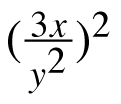b. (6x3)(3xy)–1 c.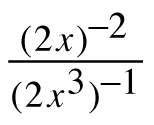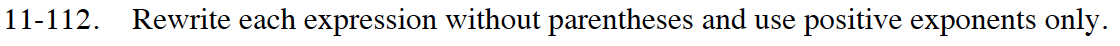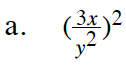Use the Power Property of Exponents and simplify.

$\frac{\left3^{1\cdot 2}\cdot x^{1\cdot 2}\right}{y^{2\cdot 2}}$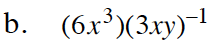Rewrite as a fraction.
Use Giant Ones to simplify.

$\frac{6x^3}{3xy}$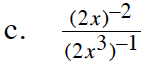Take the reciprocal of both the numerator and the denominator. Use the Power Property in the denominator, then use Giant Ones to simplify.

$\frac{2x^3}{\left(2x\right)^2}=\frac{2x^3}{4x^2}$